# Source alignment and coordinate frames#

This tutorial shows how to visually assess the spatial alignment of MEG sensor locations, digitized scalp landmark and sensor locations, and MRI volumes. This alignment process is crucial for computing the forward solution, as is understanding the different coordinate frames involved in this process.

```import numpy as np
import nibabel as nib
from scipy import linalg

import mne
from mne.io.constants import FIFF

data_path = mne.datasets.sample.data_path()
subjects_dir = data_path / "subjects"
raw_fname = data_path / "MEG" / "sample" / "sample_audvis_raw.fif"
trans_fname = data_path / "MEG" / "sample" / "sample_audvis_raw-trans.fif"
src = mne.read_source_spaces(subjects_dir / "sample" / "bem" / "sample-oct-6-src.fif")

# Load the T1 file and change the header information to the correct units
t1w = nib.load(data_path / "subjects" / "sample" / "mri" / "T1.mgz")
t1w = nib.Nifti1Image(t1w.dataobj, t1w.affine)
t1_mgh = nib.MGHImage(t1w.dataobj, t1w.affine)
```
```Opening raw data file /home/circleci/mne_data/MNE-sample-data/MEG/sample/sample_audvis_raw.fif...
Read a total of 3 projection items:
PCA-v1 (1 x 102)  idle
PCA-v2 (1 x 102)  idle
PCA-v3 (1 x 102)  idle
Range : 25800 ... 192599 =     42.956 ...   320.670 secs
Computing patch statistics...
[done]
Computing patch statistics...
[done]
```

## Understanding coordinate frames#

For M/EEG source imaging, there are three coordinate frames must be brought into alignment using two 3D transformation matrices that define how to rotate and translate points in one coordinate frame to their equivalent locations in another. The three main coordinate frames are:

• “meg”: the coordinate frame for the physical locations of MEG sensors

• “mri”: the coordinate frame for MRI images, and scalp/skull/brain surfaces derived from the MRI images

• “head”: the coordinate frame for digitized sensor locations and scalp landmarks (“fiducials”)

Each of these are described in more detail in the next section.

A good way to start visualizing these coordinate frames is to use the `mne.viz.plot_alignment` function, which is used for creating or inspecting the transformations that bring these coordinate frames into alignment, and displaying the resulting alignment of EEG sensors, MEG sensors, brain sources, and conductor models. If you provide `subjects_dir` and `subject` parameters, the function automatically loads the subject’s Freesurfer MRI surfaces. Important for our purposes, passing `show_axes=True` to `plot_alignment` will draw the origin of each coordinate frame in a different color, with axes indicated by different sized arrows:

• shortest arrow: (R)ight / X

• medium arrow: forward / (A)nterior / Y

• longest arrow: up / (S)uperior / Z

Note that all three coordinate systems are RAS coordinate frames and hence are also right-handed coordinate systems. Finally, note that the `coord_frame` parameter sets which coordinate frame the camera should initially be aligned with. Let’s have a look:

```fig = mne.viz.plot_alignment(
raw.info,
trans=trans,
subject="sample",
subjects_dir=subjects_dir,
show_axes=True,
dig=True,
eeg=[],
meg="sensors",
coord_frame="meg",
mri_fiducials="estimated",
)
mne.viz.set_3d_view(fig, 45, 90, distance=0.6, focalpoint=(0.0, 0.0, 0.0))
print(
"Distance from head origin to MEG origin: %0.1f mm"
)
print(
"Distance from head origin to MRI origin: %0.1f mm"
% (1000 * np.linalg.norm(trans["trans"][:3, 3]))
)
dists = mne.dig_mri_distances(raw.info, trans, "sample", subjects_dir=subjects_dir)
print(
"Distance from %s digitized points to head surface: %0.1f mm"
% (len(dists), 1000 * np.mean(dists))
)
```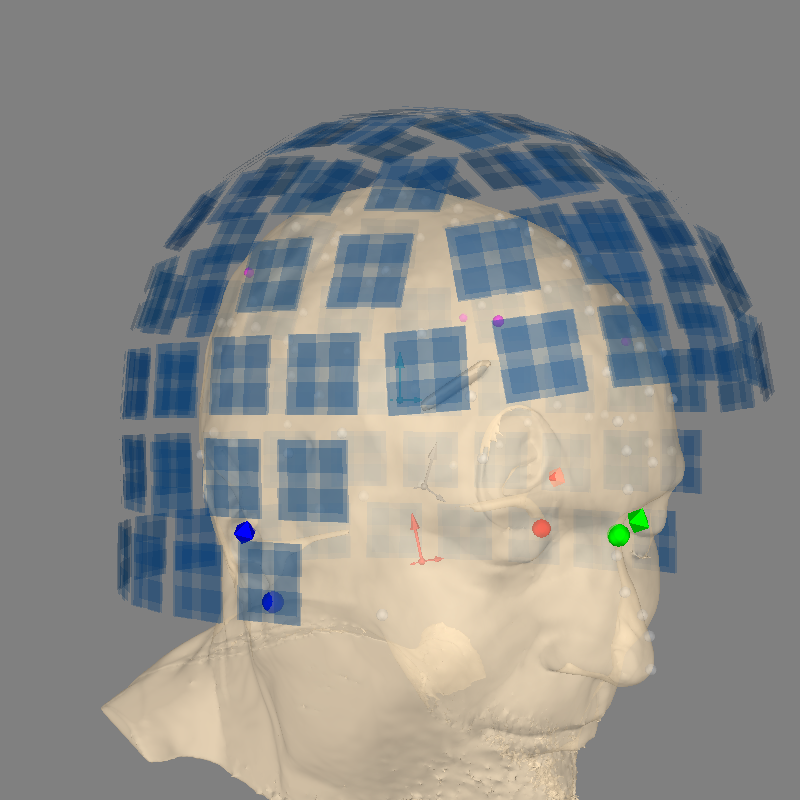```Using lh.seghead for head surface.
Channel types:: grad: 203, mag: 102
Distance from head origin to MEG origin: 65.0 mm
Distance from head origin to MRI origin: 29.9 mm
Distance from 72 digitized points to head surface: 1.7 mm
```

### Coordinate frame definitions#

The head coordinate frame is defined through the coordinates of anatomical landmarks on the subject’s head: usually the Nasion (NAS), and the left and right preauricular points (LPA and RPA). Different MEG manufacturers may have different definitions of the head coordinate frame. A good overview can be seen in the FieldTrip FAQ on coordinate systems.

For Neuromag/Elekta/MEGIN, the head coordinate frame is defined by the intersection of

1. the line between the LPA (red sphere) and RPA (purple sphere), and

2. the line perpendicular to this LPA-RPA line one that goes through the Nasion (green sphere).

The axes are oriented as X origin→RPA, Y origin→NAS, Z origin→upward (orthogonal to X and Y).

Note

The required 3D coordinates for defining the head coordinate frame (NAS, LPA, RPA) are measured at a stage separate from the MEG data recording. There exist numerous devices to perform such measurements, usually called “digitizers”. For example, see the devices by the company Polhemus.

2. MEG device coordinate frame (“meg”, blue axes)

The MEG device coordinate frame is defined by the respective MEG manufacturers. All MEG data is acquired with respect to this coordinate frame. To account for the anatomy and position of the subject’s head, we use so-called head position indicator (HPI) coils. The HPI coils are placed at known locations on the scalp of the subject and emit high-frequency magnetic fields used to coregister the head coordinate frame with the device coordinate frame.

From the Neuromag/Elekta/MEGIN user manual:

The origin of the device coordinate system is located at the center of the posterior spherical section of the helmet with X axis going from left to right and Y axis pointing front. The Z axis is, again normal to the plane with positive direction up.

Note

The HPI coils are shown as magenta spheres. Coregistration happens at the beginning of the recording and the head↔meg transformation matrix is stored in `raw.info['dev_head_t']`.

3. MRI coordinate frame (“mri”, gray axes)

Defined by Freesurfer, the “MRI surface RAS” coordinate frame has its origin at the center of a 256×256×256 1mm anisotropic volume (though the center may not correspond to the anatomical center of the subject’s head).

Note

We typically align the MRI coordinate frame to the head coordinate frame through a rotation and translation matrix, that we refer to in MNE as `trans`.

Let’s try using `plot_alignment` by making `trans` the identity transform. This (incorrectly!) equates the MRI and head coordinate frames.

```identity_trans = mne.transforms.Transform("head", "mri")
mne.viz.plot_alignment(
raw.info,
trans=identity_trans,
subject="sample",
src=src,
subjects_dir=subjects_dir,
dig=True,
coord_frame="meg",
)
```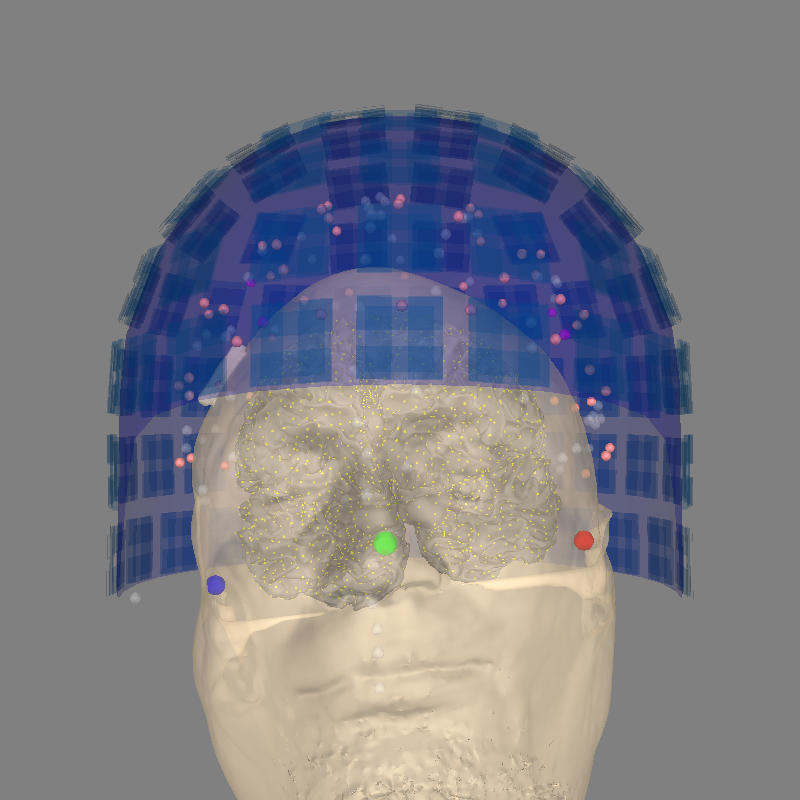```Using lh.seghead for head surface.
Getting helmet for system 306m
Channel types:: grad: 203, mag: 102, eeg: 59
```

### A good example#

Here is the same plot, this time with the `trans` properly defined (using a precomputed transformation matrix).

```mne.viz.plot_alignment(
raw.info,
trans=trans,
subject="sample",
src=src,
subjects_dir=subjects_dir,
dig=True,
coord_frame="meg",
)
```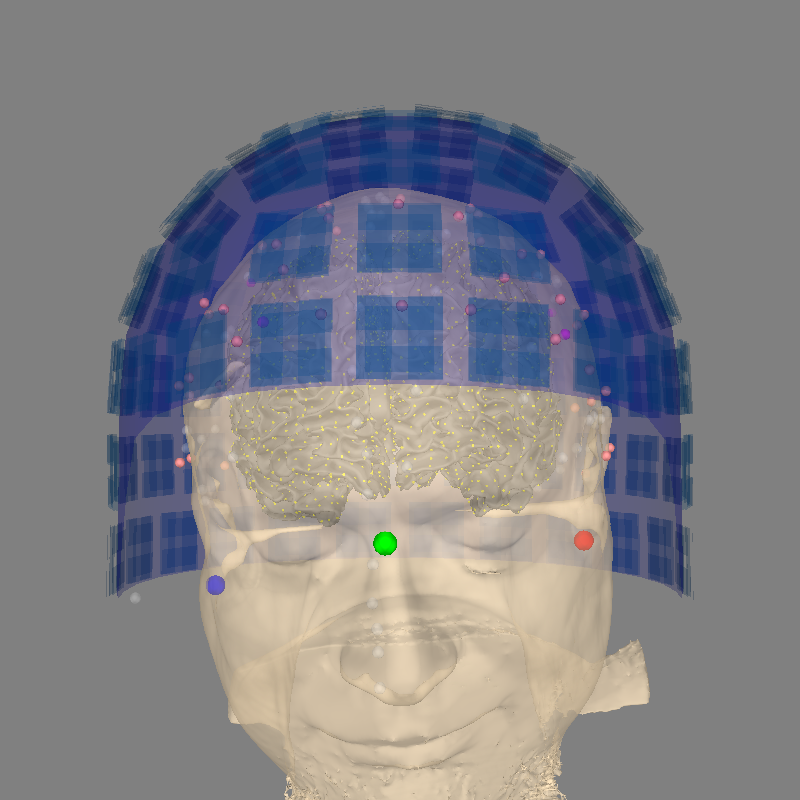```Using lh.seghead for head surface.
Getting helmet for system 306m
Channel types:: grad: 203, mag: 102, eeg: 59
```

## Visualizing the transformations#

Let’s visualize these coordinate frames using just the scalp surface; this will make it easier to see their relative orientations. To do this we’ll first load the Freesurfer scalp surface, then apply a few different transforms to it. In addition to the three coordinate frames discussed above, we’ll also show the “mri_voxel” coordinate frame. Unlike MRI Surface RAS, “mri_voxel” has its origin in the corner of the volume (the left-most, posterior-most coordinate on the inferior-most MRI slice) instead of at the center of the volume. “mri_voxel” is also not an RAS coordinate system: rather, its XYZ directions are based on the acquisition order of the T1 image slices.

```# The head surface is stored in "mri" coordinate frame
# (origin at center of volume, units=mm)
subjects_dir / "sample" / "surf" / "lh.seghead"
)

# To put the scalp in the "head" coordinate frame, we apply the inverse of
# the precomputed `trans` (which maps head → mri)

# To put the scalp in the "meg" coordinate frame, we use the inverse of
scalp_pts_in_meg_coord = mne.transforms.apply_trans(
)

# The "mri_voxel"→"mri" transform is embedded in the header of the T1 image
# file. We'll invert it and then apply it to the original `seghead_rr` points.
# No unit conversion necessary: this transform expects mm and the scalp surface
# is defined in mm.
mri_to_vox = linalg.inv(vox_to_mri)
```

Now that we’ve transformed all the points, let’s plot them. We’ll use the same colors used by `plot_alignment` and use green for the “mri_voxel” coordinate frame:

```def add_head(renderer, points, color, opacity=0.95):

renderer = mne.viz.backends.renderer.create_3d_figure(
size=(600, 600), bgcolor="w", scene=False
)
mne.viz.set_3d_view(
figure=renderer.figure,
distance=800,
focalpoint=(0.0, 30.0, 30.0),
elevation=105,
azimuth=180,
)
renderer.show()
```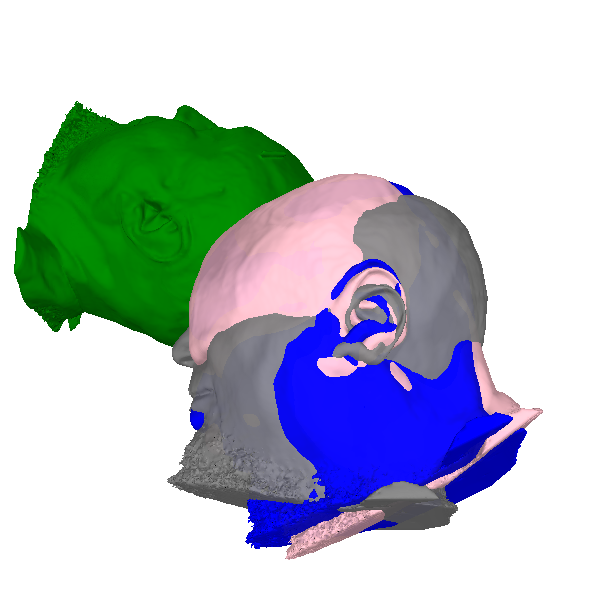The relative orientations of the coordinate frames can be inferred by observing the direction of the subject’s nose. Notice also how the origin of the mri_voxel coordinate frame is in the corner of the volume (above, behind, and to the left of the subject), whereas the other three coordinate frames have their origin roughly in the center of the head.

### Example: MRI defacing#

For a real-world example of using these transforms, consider the task of defacing the MRI to preserve subject anonymity. If you know the points in the “head” coordinate frame (as you might if you’re basing the defacing on digitized points) you would need to transform them into “mri” or “mri_voxel” in order to apply the blurring or smoothing operations to the MRI surfaces or images. Here’s what that would look like (we’ll use the nasion landmark as a representative example):

```# Get the nasion:
nasion = [
p
for p in raw.info["dig"]
if p["kind"] == FIFF.FIFFV_POINT_CARDINAL and p["ident"] == FIFF.FIFFV_POINT_NASION
]
nasion = nasion["r"]  # get just the XYZ values

# Transform it from head to MRI space (recall that `trans` is head → mri)
nasion_mri = mne.transforms.apply_trans(trans, nasion, move=True)
# Then transform to voxel space, after converting from meters to millimeters
nasion_vox = mne.transforms.apply_trans(mri_to_vox, nasion_mri * 1e3, move=True)
# Plot it to make sure the transforms worked
renderer = mne.viz.backends.renderer.create_3d_figure(
size=(400, 400), bgcolor="w", scene=False
)
renderer.sphere(center=nasion_vox, color="orange", scale=10)
mne.viz.set_3d_view(
figure=renderer.figure,
distance=600.0,
focalpoint=(0.0, 125.0, 250.0),
elevation=45,
azimuth=180,
)
renderer.show()
```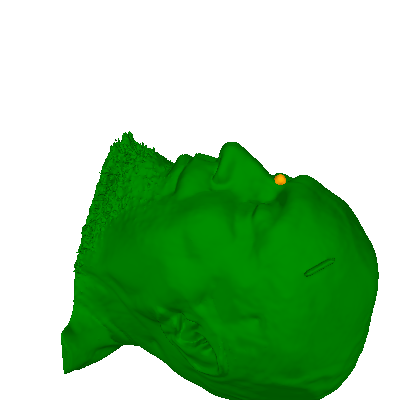## Defining the head↔MRI `trans` using the GUI#

You can try creating the head↔MRI transform yourself using `mne.gui.coregistration()`.

• To set the MRI fiducials, make sure `Lock Fiducials` is toggled off.

• Set the landmarks by clicking the radio button (LPA, Nasion, RPA) and then clicking the corresponding point in the image.

Note

The position of each fiducial used is the center of the octahedron icon.

• After doing this for all the landmarks, toggle `Lock Fiducials` radio button and optionally pressing `Save MRI Fid.` which will save to a default location in the `bem` folder of the Freesurfer subject directory.

• Then you can load the digitization data from the raw file (`Path to info`).

• Click `Fit ICP`. This will align the digitization points to the head surface. Sometimes the fitting algorithm doesn’t find the correct alignment immediately. You can try first fitting using LPA/RPA or fiducials and then align according to the digitization. You can also finetune manually with the controls on the right side of the panel.

• Click `Save` (lower right corner of the panel), set the filename and read it with `mne.read_trans()`.

Note

Coregistration can also be automated as shown in Using an automated approach to coregistration.

```mne.gui.coregistration(subject="sample", subjects_dir=subjects_dir)
```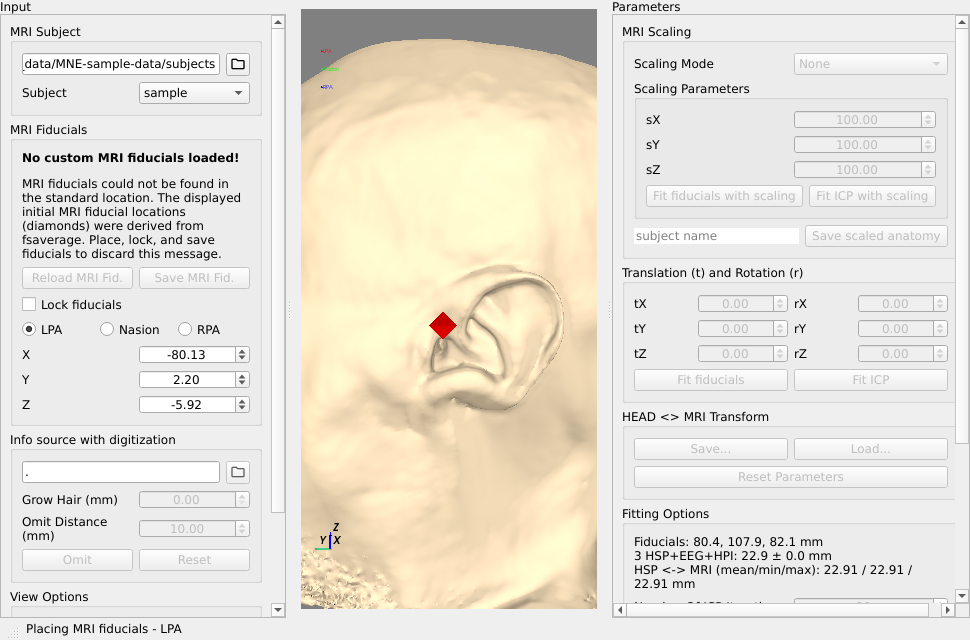```    Triangle neighbors and vertex normals...
Triangle neighbors and vertex normals...
Estimating fiducials from fsaverage.
Triangle neighbors and vertex normals...
Using fiducials from: /home/circleci/mne_data/MNE-sample-data/subjects/fsaverage/bem/fsaverage-fiducials.fif.
Triangle neighbors and vertex normals...
Triangle neighbors and vertex normals...
Estimating fiducials from fsaverage.
Estimating fiducials from fsaverage.
Placing MRI fiducials - LPA
Placing MRI fiducials - LPA
```

## Alignment without MRI#

The surface alignments above are possible if you have the surfaces available from Freesurfer. `mne.viz.plot_alignment()` automatically searches for the correct surfaces from the provided `subjects_dir`. Another option is to use a spherical conductor model. It is passed through `bem` parameter.

```sphere = mne.make_sphere_model(info=raw.info, r0="auto", head_radius="auto")
src = mne.setup_volume_source_space(sphere=sphere, pos=10.0)
mne.viz.plot_alignment(
raw.info,
trans=trans,
eeg="projected",
bem=sphere,
src=src,
dig=True,
surfaces=["brain", "inner_skull", "outer_skull", "outer_skin"],
coord_frame="meg",
show_axes=True,
)
```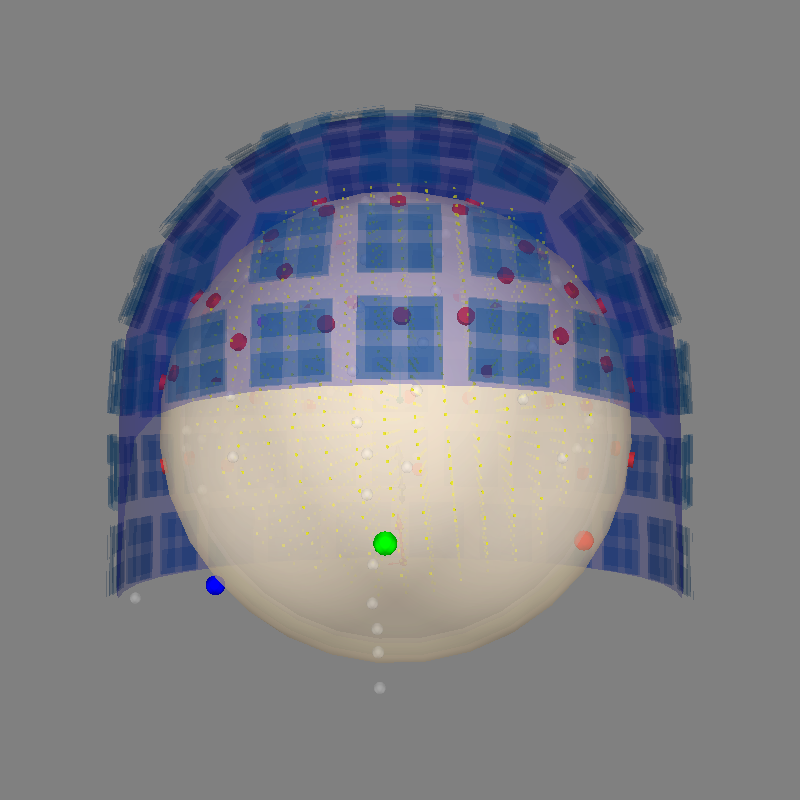```Fitted sphere radius:         91.0 mm
Origin head coordinates:      -4.1 16.0 51.7 mm
Origin device coordinates:    1.4 17.8 -10.3 mm

Equiv. model fitting -> RV = 0.00348961 %
mu1 = 0.944714    lambda1 = 0.137173
mu2 = 0.667447    lambda2 = 0.683794
mu3 = -0.26595    lambda3 = -0.0105972
Set up EEG sphere model with scalp radius    91.0 mm

Sphere                : origin at (-4.1 16.0 51.7) mm
grid                  : 10.0 mm
mindist               : 5.0 mm

Setting up the sphere...
Surface CM = (  -4.1   16.0   51.7) mm
Surface fits inside a sphere with radius   81.9 mm
Surface extent:
x =  -86.0 ...   77.8 mm
y =  -65.9 ...   97.9 mm
z =  -30.2 ...  133.7 mm
Grid extent:
x =  -90.0 ...   80.0 mm
y =  -70.0 ...  100.0 mm
z =  -40.0 ...  140.0 mm
6156 sources before omitting any.
2300 sources after omitting infeasible sources not within 0.0 - 81.9 mm.
1904 sources remaining after excluding the sources outside the surface and less than    5.0 mm inside.
Source space : MRI voxel -> MRI (surface RAS)
0.010000  0.000000  0.000000     -90.00 mm
0.000000  0.010000  0.000000     -70.00 mm
0.000000  0.000000  0.010000     -40.00 mm
0.000000  0.000000  0.000000       1.00
Getting helmet for system 306m
Channel types:: grad: 203, mag: 102, eeg: 59
Projecting sensors to the head surface
```

It is also possible to use `mne.gui.coregistration()` to warp a subject (usually `fsaverage`) to subject digitization data, see these slides.

Total running time of the script: (0 minutes 51.630 seconds)

Estimated memory usage: 636 MB

Gallery generated by Sphinx-Gallery